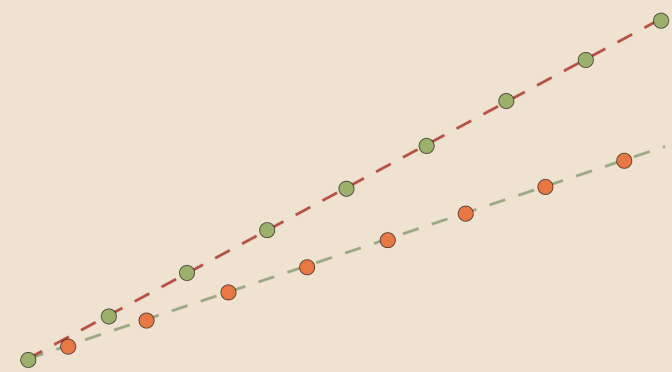Counterexamples on real sequences (part 2)

In that article, I provide basic counterexamples on sequences convergence. I follow on here with some additional and more advanced examples.

If $$(u_n)$$ converges then $$(\vert u_n \vert )$$ converge?

This is true and the proof is based on the reverse triangle inequality: $$\bigl| \vert x \vert – \vert y \vert \bigr| \le \vert x – y \vert$$. However the converse doesn’t hold. For example, the sequence $$u_n=(-1)^n$$ is such that $$\lim \vert u_n \vert = 1$$ while $$(u_n)$$ diverges.

If for all $$p \in \mathbb{N}$$ $$\lim\limits_{n \to +\infty} (u_{n+p} – u_n)=0$$ then $$(u_n)$$ converges?

The assertion is wrong. A simple counterexample is $$u_n= \ln(n+1)$$. It is well known that $$(u_n)$$ diverges. However for any $$p \in \mathbb{N}$$ we have $$\lim\limits_{n \to +\infty} (u_{n+p} – u_n) =\ln(1+\frac{p}{n+1})=0$$.
The converse proposition is true. Assume that $$(u_n)$$ is a converging sequence with limit $$l$$ and $$p \ge 0$$ is any integer. We have $$\vert u_{n+p}-u_n \vert = \vert (u_{n+p}-l)-(u_n-l) \vert \le \vert u_{n+p}-l \vert – \vert u_n-l \vert$$ and both terms of the right hand side of the inequality are converging to zero.

If $$\lim (u_{2n} – u_n) = 0$$ and $$(u_n)$$ is increasing then $$(u_n)$$ converges?

Is not true. However, in order to find a counterexample, we have to pick-up a slowly increasing sequence. As a matter of fact the sequence $$\ln(n+1)$$ is increasing too quick as $$\lim \bigl(\ln(2n+1) – \ln(n+1)\bigr) = \ln 2$$. The sequence $$u_n=\ln(\ln(n+1))$$ provides a counterexample. $$u_n$$ is increasing as the function composition of two increasing maps. We also have the equality:
$u_{2n} – u_n=\ln \left(\frac{\ln 2}{\ln(n+1)}+\frac{\ln(n+1/2)}{\ln(n+1)}\right)$ and the right end side converges to $$0$$.

If $$(u_n)$$ is positive and unbounded then $$u_n \to +\infty$$?

Does not hold. Have a look at $$u_n=\begin{cases} 0 & \text{for } n \text{ even} \\ n & \text{for } n \text{ odd} \end{cases}$$

If $$\lim u_n = +\infty$$ then $$(u_n)$$ is eventually increasing?

Still not! The sequence $$u_n=\begin{cases} n & \text{for } n \text{ even} \\ 2n & \text{for } n \text{ odd} \end{cases}$$ provides a counterexample.

If $$\lim (u_{n+1} – u_n) = 0$$ then $$\lim \frac{u_{n+1}}{u_n} = 1$$?

Is also wrong as you can see with the sequence $$u_n=\begin{cases} \frac{1}{n+1} & \text{for } n \text{ even} \\ \frac{1}{(n+1)^2} & \text{for } n \text{ odd.} \end{cases}$$

If $$\lim (u_{n+1}-u_n) =0$$ and $$(u_n)$$ is bounded then $$(u_n)$$ converges?

This is still wrong! But it is slightly more difficult to find a counterexample. One idea is to find a sequence which oscillates between $$0$$ and $$1$$ with steps decreasing to zero. The sequence:
$u_n=\begin{cases} 0 & \text{for } n = 0\\ 1-\frac{n-2^{2k}}{2^{2k}} & \text{for } 2^{2k} \le n < 2^{2k+1}\\ \frac{n-2^{2k+1}}{2^{2k+1}} & \text{for } 2^{2k+1} \le n < 2^{2k+2}\end{cases}$ is well defined and having all the required properties:

• for all $$n \in \mathbb{N}$$, $$u_n \in [0,1]$$, therefore $$(u_n)$$ is bounded.
• For $$n \ge 2^k$$ we have $$\vert u_{n+1}-u_n \vert \le \frac{1}{2^k}$$, hence $$\lim (u_{n+1}-u_n) =0$$.
• However, $$(u_n)$$ diverges as for all $$k \in \mathbb{N}$$ we have $$u_{2^{2k}}=1$$ and $$u_{2^{2k+1}}=0$$.

It is interesting to notice that the set of limit points of $$(u_n)$$ is the interval $$[0,1]$$.

The examples above were inspired from mathematical exercises given by math teacher Robert FERRÉOL.

3 thoughts on “Counterexamples on real sequences (part 2)”

1.Dan Anderson says:

Pretty sure that your counterexample for “If for all p∈N limn→+∞(un+p–un)=0 then (un) converges?” is incorrect.

By hypothesis, p is allowed to range over the entirety of N. The Archimedean property of the naturals and reals ensures there exists a p st. p > n+1 for all n (even if n→∞), and so p/(n+1) = q > 1. So, since ln is increasing, ln(1+q) > ln(1+1) = ln(2) > 0. By this method, both q and ln(1+q) can be made arbitrarily large.

Moreover, I belive that the given condition is actually equivalent to the Cauchy Criterion, and so does imply (and is implied by) the convergence of the sequence.

1.Dan Anderson says:

Also, excellent site! Don’t let the analysis-happy undergrad slow you down.